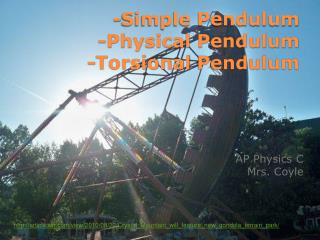DownloadDownload Presentation-Simple Pendulum -Physical Pendulum - Torsional Pendulum

# -Simple Pendulum -Physical Pendulum - Torsional Pendulum

Download Presentation## -Simple Pendulum -Physical Pendulum - Torsional Pendulum

- - - - - - - - - - - - - - - - - - - - - - - - - - - E N D - - - - - - - - - - - - - - - - - - - - - - - - - - -
##### Presentation Transcript

1. -Simple Pendulum-Physical Pendulum-Torsional Pendulum AP Physics CMrs. Coyle http://article.wn.com/view/2010/08/22/Crystal_Mountain_will_feature_new_gondola_terrain_park/

2. The motion of a simple pendulum is very close to a SHM oscillator, if the angle is <10o The Simple Pendulum

3. The tangential component of mg is the restoring force • s=Lsin q • For small values of q, sin q= q • Since αis proportional to q this motion is SHM and let ω2=g/L

4. q = qmax cos (wt + f) Angular frequency: Period: Period of the Simple Pendulum

5. Question: If a pendulum was taken to a planet where the acceleration due to gravity was four times that of g on the earth, how would the period change?

6. An object that oscillates about a fixed axis (not through its center of mass) and the object cannot be approximated as a particle. • Torque = Iα • For small angles sinθ=θ Physical Pendulum

7. Since α is proportional to θ the motion has the form of an object in simple harmonic motion. • Angular frequency: • Period: Period of the Physical Pendulum

8. TorsionalPendulum • Restoring torque t = -kq =Iα k is the torsion constant of the wire

9. Angular frequency: • Period: Period of Torsional Pendulum